# Lumped Element Wilkinson Splitters

Click here to go to our main page on Wilkinson power dividers

Content was donated by Dr. Antonije Djordjevic, a Professor at University of Belgrade. The real Belgrade, not Belgrade, Maine, by the way. Thanks, Tony!

This design below provides lumped-element equivalent of simple one-stage Wilkinson power divider with nominal port impedance 50 ohms. The center frequency was picked to be 1.592 GHz, so that angular frequency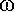=1010 radians/second. You can apply scale factors to the capacitors and inductors to change center frequency, both are inversely proportional to frequency. Note that the isolation resistor value is the same as in a "normal" Wilkinson, at 2xZ0.

The normalized inductor reactance at center frequency:

XL1=sqrt(2)

The normalized capacitor susceptances at center frequency:

BC1=sqrt(2), BC2=sqrt(2)/2

Let's review the formulas for capacitive and inductive reactance while we're on the subject: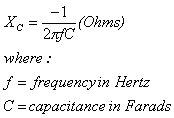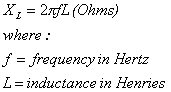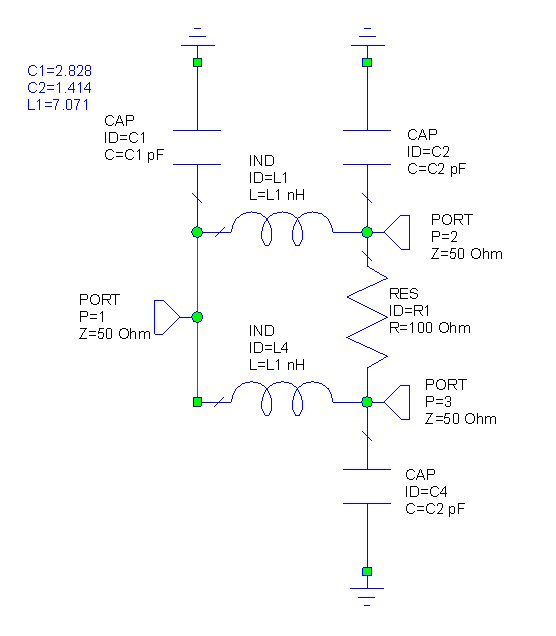Here's how it performs over frequency: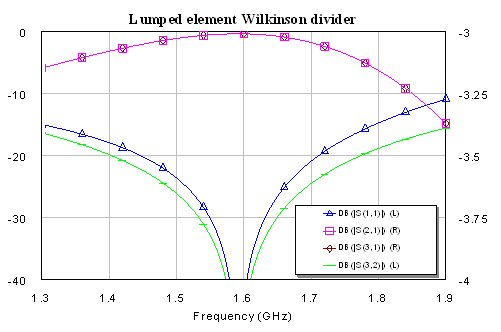Now for the derivation...

One way to derive the lumped-element equivalent of the Wilkinson power divider is to begin by deriving a lumped-element equivalent of a quarter-wave transmission line.
Suppose that the characteristic impedance of the line is ZC=Z0, where Z0 is the port nominal impedance. If the line length is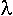/4, the matrix of scattering parameters of the line is: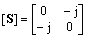We consider the pi-network of lumped elements, which consists of capacitors in shunt branches and an inductor in the series branch. We want the network to have the same scattering parameters as the transmission line. Due to the symmetry, the two capacitors must be identical (C1=C2=C). We place a generator of emf E=2V, angular frequency, and internal resistance R1=Z0 at one port. We terminate the other port in R2=Z0.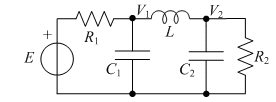Let V1 and V2 be the input and output voltages (the lower nodes are assumed grounded). To obtain the required scattering matrix, we should have complex voltages V1=1 Volt and V2=-j Volt. The nodal equations for this circuit read: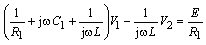and: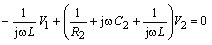Substituting V1= 1V, V2=-jV, R1=R2=Z0, and C1=C2=C into the second equation, we readily obtain jC+1/(jL)=0 andL=Z0, so thatC=1/Z0. Hence, the reactance of the coil is XL=Z0 and the susceptance of the capacitor is BC=1/Z0.

In the transmission-line Wilkinson power divider (for 50 Ohm nominal port impedances), we have two quarter-wavelength transmission lines whose characteristic impedance are 50xSQRT(2)=70.7 ohms. Each line can be replaced by a pi-network with XL=70.7 Ohms and BC=1/70.7 Siemens, which are the same values as in our scheme. (Two capacitors at port 1 are merged into an equivalent capacitor with two times larger capacitance.)

References for this work:

Djordjevic, A. R., BaÅ¾dar, M. B., VitoÅ¡evic, G. M., Sarkar, T. K., Harrington, R. F., Scattering Parameters of Microwave Networks with Multiconductor Transmission Lines (software and user's manual), Artech House, Boston, 1989.

Djordjevic, A. R., BaÅ¾dar, M. B., Harrington, R. F., Sarkar, T. K., LINPAR for Windows: Matrix Parameters for Multiconductor Transmission Lines, Version 2.0 (software and user's manual), Artech House, Boston, 1999.

Another solid gold addition to Microwaves101's knowledge base from our international audience. Dig it, dudes, and keep those emails coming!

Author : Dr. Antonije Djordjevic